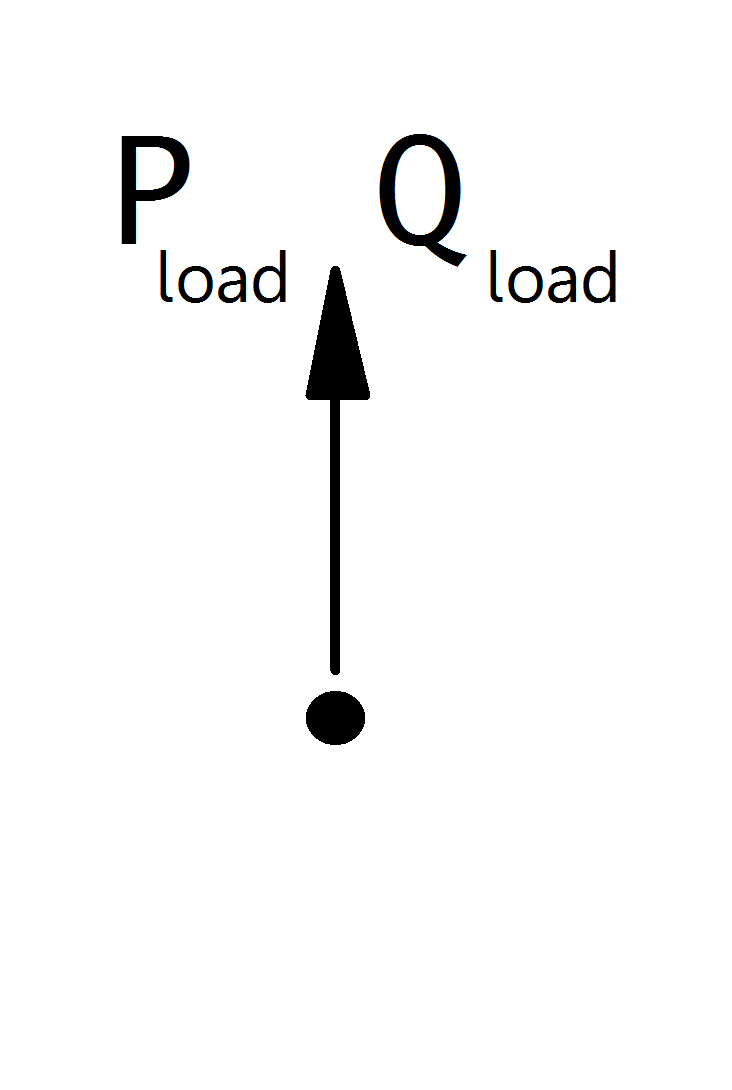# Static Generator¶

Note

Static generators should always have a positive p_mw value, since all power values are given in the generator convention. If you want to model constant power consumption, it is recommended to use a load element instead of a static generator with negative active power value. If you want to model a voltage controlled generator, use the generator element.

## Create Function¶

pandapower.create_sgen(net, bus, p_mw, q_mvar=0, sn_mva=nan, name=None, index=None, scaling=1.0, type='wye', in_service=True, max_p_mw=nan, min_p_mw=nan, max_q_mvar=nan, min_q_mvar=nan, controllable=nan, k=nan, rx=nan, current_source=True)

Adds one static generator in table net[“sgen”].

Static generators are modelled as positive and constant PQ power. This element is used to model generators with a constant active and reactive power feed-in. If you want to model a voltage controlled generator, use the generator element instead.

gen, sgen and ext_grid in the grid are modelled in the generator system! If you want to model the generation of power, you have to assign a positive active power to the generator. Please pay attention to the correct signing of the reactive power as well (positive for injection and negative for consumption).

INPUT:

net - The net within this static generator should be created

bus (int) - The bus id to which the static generator is connected

p_mw (float) - The real power of the static generator (positive for generation!)

OPTIONAL:

q_mvar (float, 0) - The reactive power of the sgen

sn_mva (float, None) - Nominal power of the sgen

name (string, None) - The name for this sgen

index (int, None) - Force a specified ID if it is available. If None, the index one higher than the highest already existing index is selected.

scaling (float, 1.) - An OPTIONAL scaling factor to be set customly

type (string, None) - Three phase Connection type of the static generator: wye/delta

in_service (boolean) - True for in_service or False for out of service

max_p_mw (float, NaN) - Maximum active power injection - necessary for controllable sgens in OPF

min_p_mw (float, NaN) - Minimum active power injection - necessary for controllable sgens in OPF

max_q_mvar (float, NaN) - Maximum reactive power injection - necessary for controllable sgens in OPF

min_q_mvar (float, NaN) - Minimum reactive power injection - necessary for controllable sgens in OPF

controllable (bool, NaN) - Whether this generator is controllable by the optimal powerflow

Defaults to False if “controllable” column exists in DataFrame

k (float, NaN) - Ratio of nominal current to short circuit current

rx (float, NaN) - R/X ratio for short circuit impedance. Only relevant if type is specified as motor so that sgen is treated as asynchronous motor

current_source (bool, True) - Model this sgen as a current source during short- circuit calculations; useful in some cases, for example the simulation of full- size converters per IEC 60909-0:2016.

OUTPUT:
index (int) - The unique ID of the created sgen
EXAMPLE:
create_sgen(net, 1, p_mw = -120)
pandapower.create_sgen_from_cosphi(net, bus, sn_mva, cos_phi, mode, **kwargs)

Creates an sgen element from rated power and power factor cos(phi).

INPUT:

net - The net within this static generator should be created

bus (int) - The bus id to which the static generator is connected

sn_mva (float) - rated power of the generator

cos_phi (float) - power factor cos_phi

mode (str) - “ind” for inductive or “cap” for capacitive behaviour

OUTPUT:
index (int) - The unique ID of the created sgen

gen, sgen, and ext_grid are modelled in the generator point of view. Active power will therefore be postive por generation, and reactive power will be negative for consumption behaviour and positive for generation behaviour.

## Input Parameters¶

net.sgen

 Parameter Datatype Value Range Explanation name string name of the static generator type string naming conventions: “PV” - photovoltaic system “WP” - wind power system “CHP” - combined heating and power system type of generator bus* integer index of connected bus p_mw* float $$\leq$$ 0 active power of the static generator [MW] q_mvar* float reactive power of the static generator [MVar] sn_mva float $$>$$ 0 rated power ot the static generator [MVA] scaling* float $$\geq$$ 0 scaling factor for the active and reactive power max_p_mw** float Maximum active power [MW] min_p_mw** float Minimum active power [MW] max_q_mvar** float Maximum reactive power [MVar] min_q_mvar** float Minimum reactive power [MVar] controllable** bool States if sgen is controllable or not, sgen will not be used as a flexibilty if it is not controllable k*** float $$\geq$$ 0 Ratio of nominal current to short circuit current rx*** float $$\geq$$ 0 R/X ratio for short circuit impedance. Only relevant if type is specified as motor so that sgen is treated as asynchronous motor in_service* boolean True / False specifies if the generator is in service.

*necessary for executing a power flow calculation
**optimal power flow parameter

## Electric Model¶

Static Generators are modelled as PQ-buses in the power flow calculation:The PQ-Values are calculated from the parameter table values as:

\begin{align*} P_{sgen} &= p\_mw \cdot scaling \\ Q_{sgen} &= q\_mvar \cdot scaling \\ \end{align*}

Note

The apparent power value sn_mva is provided as additional information for usage in controller or other applications based on panadapower. It is not considered in the power flow!

## Result Parameters¶

net.res_sgen

 Parameter Datatype Explanation p_mw float resulting active power demand after scaling [MW] q_mvar float resulting reactive power demand after scaling [MVar]

The power values in the net.res_sgen table are equivalent to $$P_{sgen}$$ and $$Q_{sgen}$$.